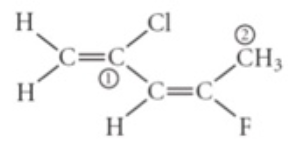# Problem: Consider the molecule below. Determine the molecular geometry at each of the 2 labeled carbons.A) C1 = bent, C2 = trigonal planar B) C1 = tetrahedral, C2 = linear C) C1 = trigonal planar, C2 = bent D) C1 = trigonal planar, C2 = tetrahedral E) C1 = trigonal pyramidal, C2 = see-saw

###### FREE Expert Solution99% (187 ratings)###### Problem Details

Consider the molecule below. Determine the molecular geometry at each of the 2 labeled carbons.A) C1 = bent, C2 = trigonal planar

B) C1 = tetrahedral, C2 = linear

C) C1 = trigonal planar, C2 = bent

D) C1 = trigonal planar, C2 = tetrahedral

E) C1 = trigonal pyramidal, C2 = see-saw